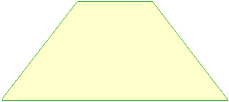Year 11. Please help me, I am stuck on what appears to be a really simple question. What am I missing? This is the problem: A trapezium has a perimeter of 22cm. The 2 parallel sides are such that the length of one is three times the length of the other. The non-parallel sides are equal. If the distance between the parallel sides is 4cm, find the lengths of the 4 sides. (Also required to find the area of the trapezium later, but I can do that part if only I can find the lengths of the sides). I've tried to use a simple equation with x: 4x + (22-4x)= 22 but of course that comes to 0 which doesn't help I've tried to use a simultaneous linear equation: 4x + 2y = 22 but I can't find a second line that makes any sense. And I can't figure out how the distance of 4cm can be used to help solve this part of the problem. WHAT am I missing? Many thanks for any help you can give. Ben Hi Ben, You are missing this picture:There is only one way to draw a trapezium with the bottom side three times the top side AND THE TWO REMAINING SIDES EQUAL. Can you see why? Now you should find the length of the sides with the help of the pythagorean theorem. Cheers, Claude Go to Math Central